# Linear Equations In Two Variables NCERT Solutions : Class 9 Maths

## NCERT Solutions for Class 9 Maths : Linear Equations In Two VariablesFilter
Filters :
Classes
•
•
•
•
•
•
•
Chapters
•
•
•
•
•
•
•
•
•
•
• 7 More
Exercises
•
•
•
•
•

### NCERT Class 9 | LINEAR EQUATIONS IN TWO VARIABLES | Exercise 03 | Question No. 04

The taxi fare in a city is as follows: For the first kilometre, the fare is Rs 8 and for the subsequent distance it is Rs 5 per km. Taking the distance covered as x km and total fare as Rs y, write a linear equation for this information, and draw its graph.### NCERT Class 9 | LINEAR EQUATIONS IN TWO VARIABLES | Exercise 03 | Question No. 05

From the choices given below, choose the equation whose graphs are given in Fig. 4.6 and Fig. 4.7. For Fig.4.6 (i) y=x (ii) x+y=0 (iii) y=2x (iv) 2+3y=7x For Fig.4.7. (i) y=x+2 (ii) y=x-2 (iii) y=-x+2 (iv) `x+2### NCERT Class 9 | LINEAR EQUATIONS IN TWO VARIABLES | Exercise 03 | Question No. 06

If the work done by a body on application of a constant force is directly proportional to the distance travelled by the body, express this in the form of an equation in two variables and draw the graph of the same by taking the constant force as 5 units. Also read from the graph the work done when the distance travelled by the body is (i) 2 units          (ii) 0 unit### NCERT Class 9 | LINEAR EQUATIONS IN TWO VARIABLES | Exercise 03 | Question No. 07

Yamini and Fatima, two students of Class IX of a school, together contributed Rs 100 towards the Prime Minister's Relief Fund to help the earthquake victims. Write a linear equation which satisfies this data. (You may take their contributions as Rs x and Rs y.) Draw the graph of the same.Doubtnut one of the best online education platform provides free NCERT Solutions of Maths for Class 9 Linear Equations In Two Variables which are solved by our maths experts as per the NCERT (CBSE) guidelines. We provide the solutions as video solutions in which concepts are also explained along with answer which will help students while learning , preparing for exams and in doing homework. These Solutions will help to revise complete syllabus and score more marks in examinations.

Get here free, accurate and comprehensive NCERT Solutions for Class 9 Maths Linear Equations In Two Variables which have been reviewed by our maths counsellors as per the latest edition following up the CBSE guidelines. We provide video solutions in which solutions to all the questions of NCERT Class 9 Maths textbook are explained in step by step and detailed way.

## The Topics of the Chapter Linear Equations In Two Variables are :

GRAPH OF A LINEAR EQUATION IN TWO VARIABLES

SOLUTION OF A LINEAR EQUATION IN TWO VARIABLES

RECTANGULAR AND CARTESIAN COORDINATE OF A POINT

INTRODUCTION

It contains these exercises along with solved examples. All the exercises are solved in the video. Select the exercise to view the solutions exercisewise of the Chapter Linear Equations In Two Variables:

## NCERT Solutions Class 9 Maths Chapter Linear Equations In Two Variables Exercises:

We have covered all the exercises and also Solved examples in the videos. Along with the practise exercise Students should also practise solved examples to clear the concepts of the Linear Equations In Two Variables. If incase you have any doubt you can watch the solutions for the given questions.Watch the solutions for the given questions in which it is also explained in the video steps to solve the questions along with answers.

# NCERT Class 9 Solutions Chapter 4 Linear Equations in Two Variables

NCERT books have been one of the most preferred textbooks in India by the students studying the CBSE syllabus. The students find the concepts really easy to understand when learned from NCERT books, especially a subject like Maths. This is owing to the reason that NCERT books provide an easy explanation to even the difficult concepts giving various examples related to real life. The step-by-step approach followed to solve the problems is the highlight of the textbook and it makes the process of study easy for the learners.

In class 9 Maths, through the previous units, the concepts of Linear Equations in One Variable were covered in-depth in the NCERT book. By now, the students must have got a sound knowledge about the definition of linear equations and solutions of linear equation in one variable. Now in this chapter, students will study important topics of linear equations in two variables. All the vital concepts in this chapter are well explained in NCERT books along with plenty of examples and exercises.

NCERT books cover all that is required to face not just the CBSE board school exams but also aids in cracking the other competitive entrance examinations. The more you practice the more you master in maths. For a subject like Maths, it is essential that the learners practice more and more problems in order to learn the concepts of a chapter comprehensively. The NCERT textbook provides an ample number of problems that are sufficient for the students' practice. Now with a proper textbook for reference, the next important requirement for the students would be a good solution guide to the exercise questions from the textbook. The solutions guide proves to be very useful when the students try to solve the difficult textbook questions. With the help of a proper solutions guide, the students who are into self-study would benefit a lot.

At Doubtnut, an online learning platform, we provide all the solutions worked out for the NCERT textbook, exercise wise. NCERT Class 9 Solutions Chapter 4 Linear Equations in Two Variables are presented in the form of videos that can be accessed from anywhere at any time. The students can rely on these solution videos as all problems, including the complex ones are broken down into simpler solutions by our experts who are available to solve students queries whenever they require. Theories, as well as exercises of the chapter, are available at our website and if students download the Doubtnut app, it becomes even easier and convenient for them to pass the examination with flying colors.

## Linear equation in two variables – Class 9 Maths NCERT Solutions

In previous chapters, students have learned about linear equation in one variable and their different properties.  Now further in this chapter students will be learning about linear equations in two variables, how to represent the linear equation in two variables on a graph, etc. Let us recall and study the chapter in detail now.

### What are linear equations in one variable?

An equation that can be written in form of ax b c + =where a, b and c are real numbers then these equations are called linear equations in one variable and these are called first degree equations as exponent or power of the variable is considered to be one (1)

Example - 3x = 2x + x

### What are linear equations in two variables?

An equation which can be written in the form of ax+by+c =0 where a, b and c are real numbers and a, b are not equal to zero then it is called linear equation in two variables where x and y are the two variables. The solution of such types of equations is given by pair of values, one for x and other for y which makes both sides of the equation equal.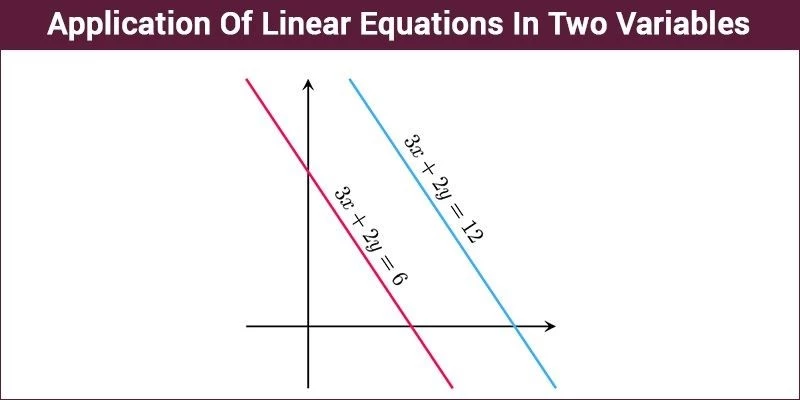Example of linear equations in two variables –

5x + 3y = 30

The above equation has two variables namely x and y.

Graphically this equation can be represented by substituting the variables to zero.

The value of x when y=0 is

5x + 3(0) = 30

x = 6

and the value of y when x = 0 is,

5 (0) + 3y = 30

y = 10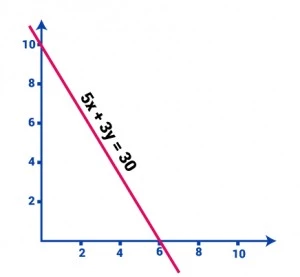Therefore, we can conclude that to solve linear equation in two variables, two equations have to be known and then the substitution method can be followed.

## Topics and Subtopics of NCERT Class 9 Maths Chapter 4 – Linear Equations In Two Variables

NCERT Class 9 Chapter 4, Linear Equations in Two Variables, is divided into further topics and subtopics in which the content of previous knowledge of the student is given as well as the current syllabus is covered in form of theory and exercises. Knowing the topics and subtopics of a given chapter helps the learners be aware of the whole content in a short form. The Knowledge of topics and subtopics help students to plan their studies accordingly in an organized manner. Let us look at the topics and Subtopics of NCERT Class 9 Maths Chapter 6 – Linear equations in two variables, according to which the solutions are worked out on the Doubtnut mobile app and website:

### Solutions of system of linear equations in two variables

As we know that linear equation in one variable has a unique solution but for the solution of linear equation in two variables, we need pair of values one for x and one for y which can satisfy both sides of equations.

Basically, there are three types of system of a linear equation and they have three types of solutions-

#### Independent system

In this system, we have exactly one pair of solution (x, y). If we represent it through the graph, the solution is the point where the two lines intersect.

#### Inconsistent system

In this system, the equation has no solution. When represented through graph the two lines will be parallel and never intersect.

#### Dependent system

In this system, the equation has infinitely many solutions. In graphical representations, the lines are coincident; they are the same line, so every coordinate pair on the line is a solution to both equations.

In below figure graphical representation of all the three systems is explained –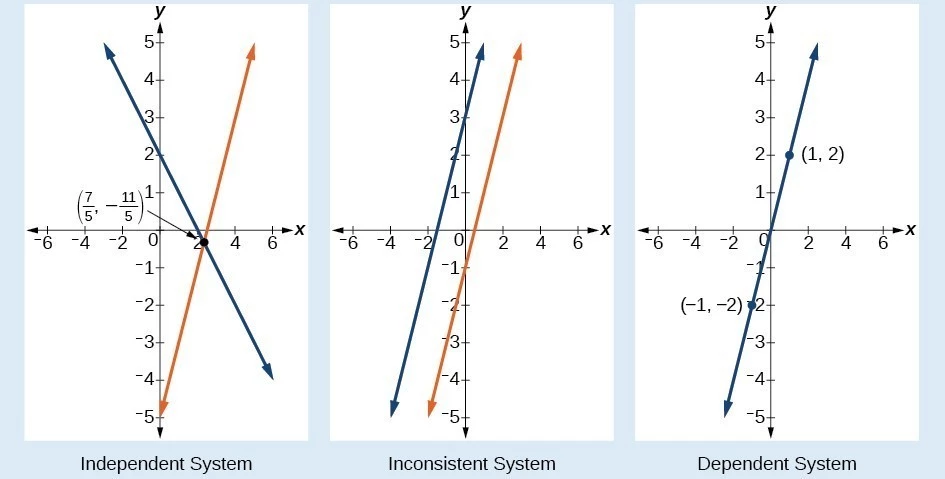### Graphical representation of linear equations in two variables

So far, we have learned to obtain the solutions of linear equation in two variables in algebraic form. We know that some equations have many solutions. Now we will learn how to represent it on the graph.

Graphs are the most useful tools in mathematics for conveying information in a meaningful way. It is usually a very helpful method for those who cannot understand the numerical terms and equations easily. Even those who may not be mathematically inclined can take solace in the basic elegance of a two-dimensional graph representing the relationship between a pair of variables.

Linear equation with two variables can be written in the form Ax + By = C and the resulting graph is always a straight line. We can also see the equation takes the form y = mx + b, where m is the slope of the line of the corresponding graph and b is its y-intercept, the point at which the line meets the y-axis.

The graph of a linear equation in two variables is a line (that's why they call it linear).

If you know an equation is linear, you can graph it by finding any two solutions

(x1, y1)(x1, y1) and (x2, y2)(x2, y2) ,

Plotting these two points, and drawing the line connecting them.

Example 1:

Graph the equation x+2y=7.

We can find two solutions, corresponding to the xx-intercepts and yy-intercepts of the graph, by setting first x=0x=0 and then y=0y=0.

When x=0 ,

we get:

0+2y=7

y=3.50

When y=0,

we get:

x+2(0)=7

x = 7

So the two points are (0,3.5)(0,3.5) and (7,0)(7,0) .

Plot these two points and draw the line connecting them.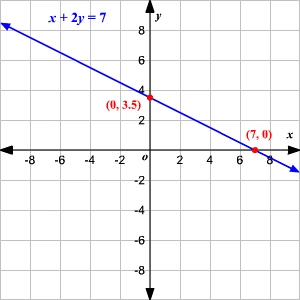If the equation is in slope-intercept form or point-slope form, we can also use the slope to help in the graph.

Example 2:

Horizontal line: y=3

Vertical line: x=−2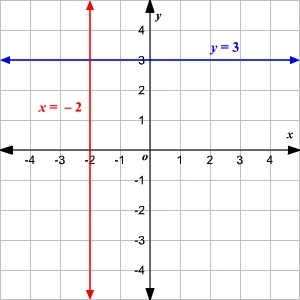## Significance of NCERT Mathematics Book Class 9

1. NCERT books are loaded with the study material as per CBSE curriculum. An ample number of exercises and examples are given of each and every topic which helps students to understand and learn the concepts in an easy and convenient language.
2. Numbers of examples are given based on daily life activities in NCERT books that help students to understand the concept in an easy way.
3. The book contains in-depth explanations of all the important concepts, which help students to understand the concepts in a better way.
4. Different methods and concepts in this book are explained in an easy to understand language that makes it quite helpful for all the students.
5. Ample of graphical representation and exercise on the same are illustrated in NCERT book of the given chapter to make the concept clearer to the students.

The students are benefitted while practicing from the NCERT book and also if they have further doubts it can be cleared with the help of online video-tutorials which are given on our website where the experts are available to help them in every possible way. It might sound difficult in the beginning, but with the help of right study material the students can easily master even the complex concepts. At doubtnut.com, all of these concepts are taught in a video format and also presented in pdf format.  It helps students to learn the lessons with better concentration with comfort of their home. The lessons taught in a step-by-step manner by an expert teacher helps in understanding the concepts in a better way. All the doubts occurring during the learning process can be easily clarified by just posting the picture of the question on the Doubtnut app or website. Thus, Doubtnut is the right platform and students should download our app for easy access to their queries. Our website makes the perfect tutorial platform for the students to face both board exams and the competitive exams in a fun way!

Share this on :-